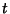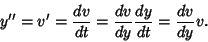## Autonomous

A differential equation or system of Ordinary Differential Equations is said to be autonomous if it does not explicitly contain the independent variable (usually denoted). A second-order autonomous differential equation is of the form, where. By the Chain Rule,can be expressed asFor an autonomous ODE, the solution is independent of the time at which the initial conditions are applied. This means that all particles pass through a given point in phase space. A nonautonomous system offirst-order ODEs can be written as an autonomous system ofODEs by lettingand increasing the dimension of the system by 1 by adding the equation